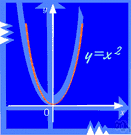# single-valued function

(redirected from Single-valued)
Also found in: Thesaurus, Encyclopedia.
ThesaurusAntonymsRelated WordsSynonymsLegend:
 Noun 1single-valued function - (mathematics) a mathematical relation such that each element of a given set (the domain of the function) is associated with an element of another set (the range of the function)multinomial, polynomial - a mathematical function that is the sum of a number of termsmath, mathematics, maths - a science (or group of related sciences) dealing with the logic of quantity and shape and arrangementmathematical relation - a relation between mathematical expressions (such as equality or inequality)expansion - a function expressed as a sum or product of terms; "the expansion of (a+b)^2 is a^2 + 2ab + b^2"inverse function - a function obtained by expressing the dependent variable of one function as the independent variable of another; f and g are inverse functions if f(x)=y and g(y)=xKronecker delta - a function of two variables i and j that equals 1 when i=j and equals 0 otherwisemetric, metric function - a function of a topological space that gives, for any two points in the space, a value equal to the distance between themtransformation - (mathematics) a function that changes the position or direction of the axes of a coordinate systemisometry - a one-to-one mapping of one metric space into another metric space that preserves the distances between each pair of points; "the isometries of the cube"operator - (mathematics) a symbol or function representing a mathematical operationcircular function, trigonometric function - function of an angle expressed as a ratio of the length of the sides of right-angled triangle containing the anglethreshold function - a function that takes the value 1 if a specified function of the arguments exceeds a given threshold and 0 otherwiseexponential, exponential function - a function in which an independent variable appears as an exponent
References in periodicals archive ?
We define a new weighted IN-cross entropy measure in the single-valued neutrosophic set environment and prove its basic properties.
To practice NSs in real life situations efficiently,The subclass of the neutrosophic sets called single-valued neutrosophic set (in short SVNS) was defined by Smarandache in .
o] : A [right arrow] P(R) is a single-valued mapping function from a task to its set of output relevant-data variables, and [[rho].
For simplicity and practical application, Wang proposed the single-valued NS (SVNS) and the interval-valued NS (IVNS) which are the instances of NS and gave some operations on these sets [8, 9].
17] introduced the concept of single-valued neutrosophic set (SVNS), a subclass of the neutrosophic sets.
Ciric et all  proved fixed point theorems for single-valued mappings, extended to a coincid ence theorems for a pair of a random operator f : X X and a multi-valued random operator T : X CB( X ) .
In the context of operational hydrologic forecasting in the NWS, the HEFS has been developed to improve upon operational single-valued forecasting and seasonal ESP forecasting while capturing user requirements, which include 1) supporting both real-time ensemble forecasting and hindcasting for large-sample verification and systematic evaluation, 2) maintaining interoperability with the single-valued forecasting system for the short range (given that single-valued forecasting is only a special case of ensemble forecasting), and 3) producing ensemble forecast information that is statistically consistent over a wide range of spatiotemporal scales.
According to the drilling risk mode: (1) In figure 2 (a), Because of the single-valued pressure curve, the formation pressure is also a single value.
The study is qualitative in that it reports on these teacher trainees' mental constructions of the concept of continuity of single-valued functions, obtained from analysis of their responses to structured activity sheets.
Sustainability is not a single-valued function; rather, sustainability is a holistic property of a system.
Hybrid fixed point theory is a recent development is the ambit of fixed point theorems for contracting single-valued and multivalued maps in metric spaces.
For a given m-accretive mapping A, B, C, G: E [right arrow], CB(E), nonlinear mappings N(x,x), M(x,x): E x E [right arrow] E, single-valued mapping g; H [right arrow], H, any given f [member of] E and [lambda] > 0, find u [member of] E, [bar.

Site: Follow: Share:
Open / Close